# RS Aggarwal Solutions for Class 6 Maths Chapter 4 Integers

## Class 6 RS Aggarwal Chapter 4 – Integers

RS Aggarwal Solutions for Class 6 Maths, Chapter 4 are provided here. Students can refer the RS Aggarwal Solutions for Class 6 Chapter 4 to prepare well for the exam and improve their academic performance. To score good marks in examination, students need to practice RS Aggarwal Solutions for Class 6 Maths. Solving textbook questions will help the students to understand the topics discussed in this chapter and also increase their self-confidence.

Students can refer the RS Aggarwal Solutions for Class 6 Chapter 4 in pdf format which has been solved by the experts at BYJUS. Download pdf of Class 6 Chapter 4 in their respective links.

## Download PDF of RS Aggarwal Solutions for Class 6 Maths Chapter 4 Integers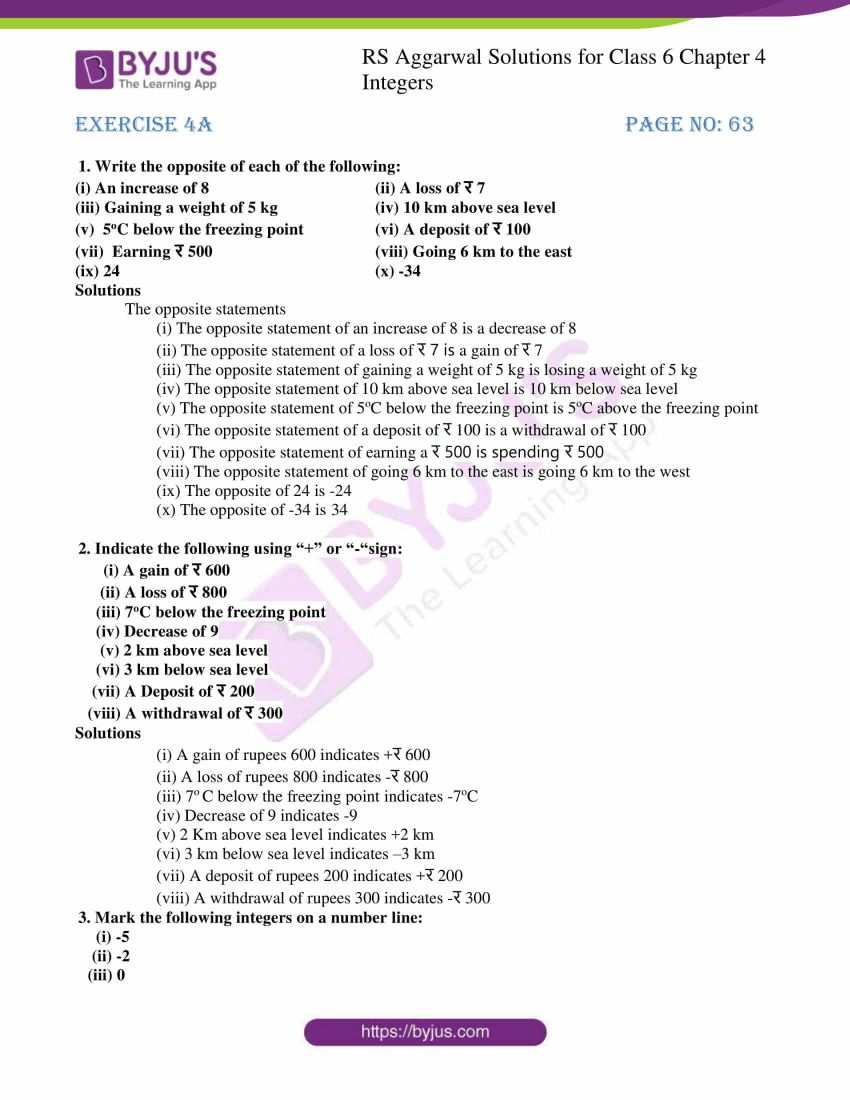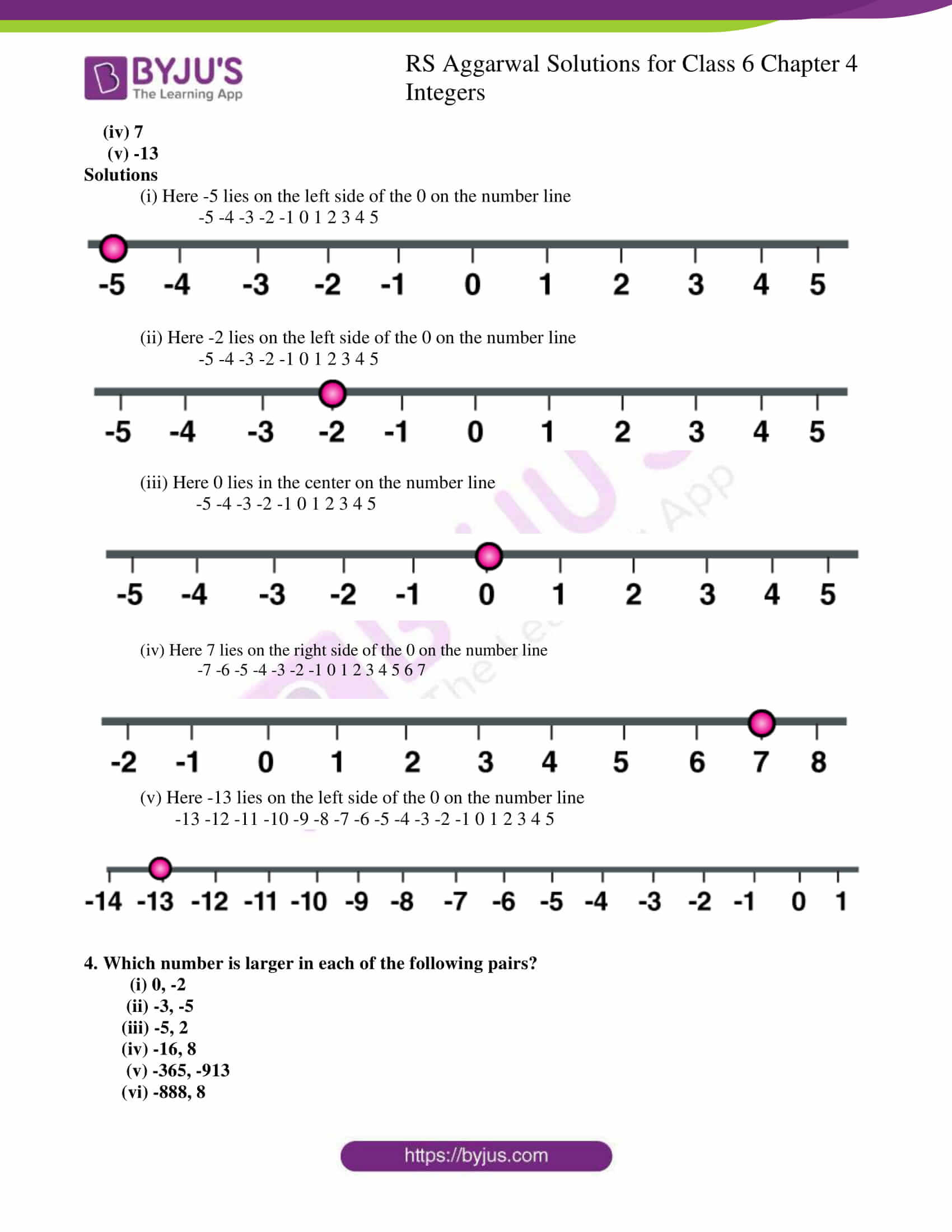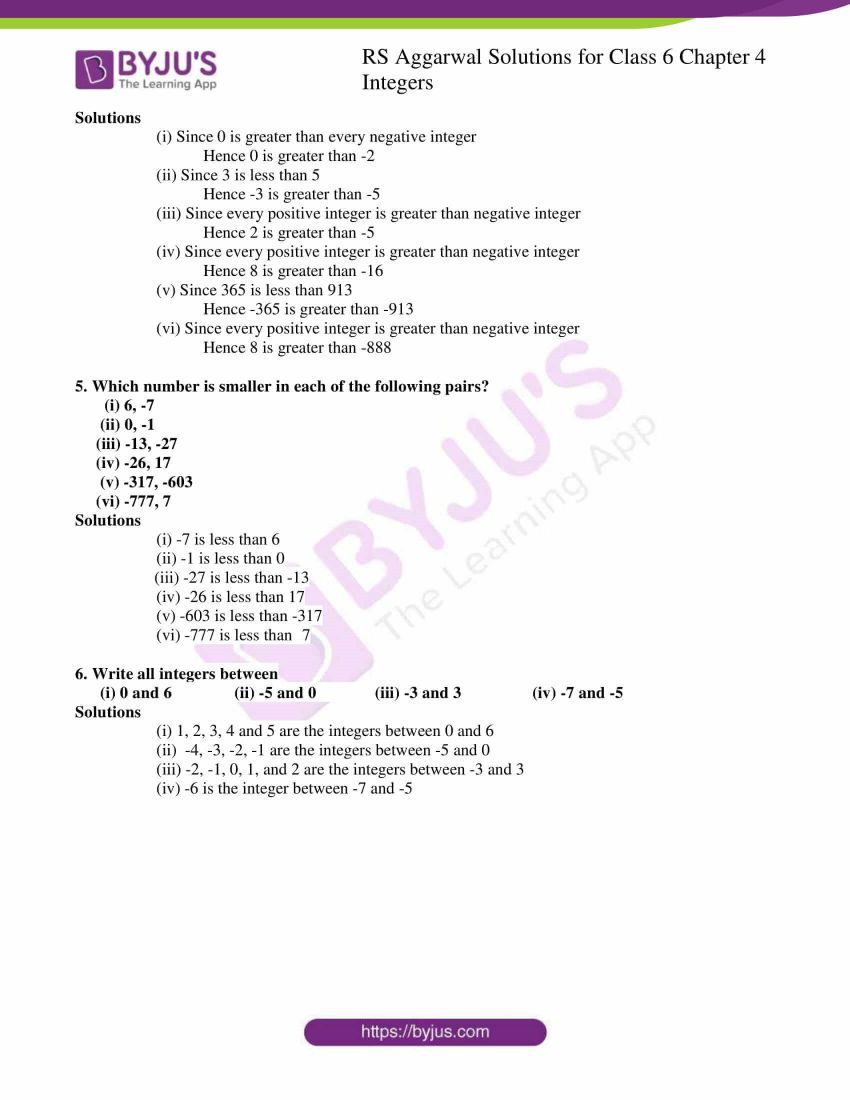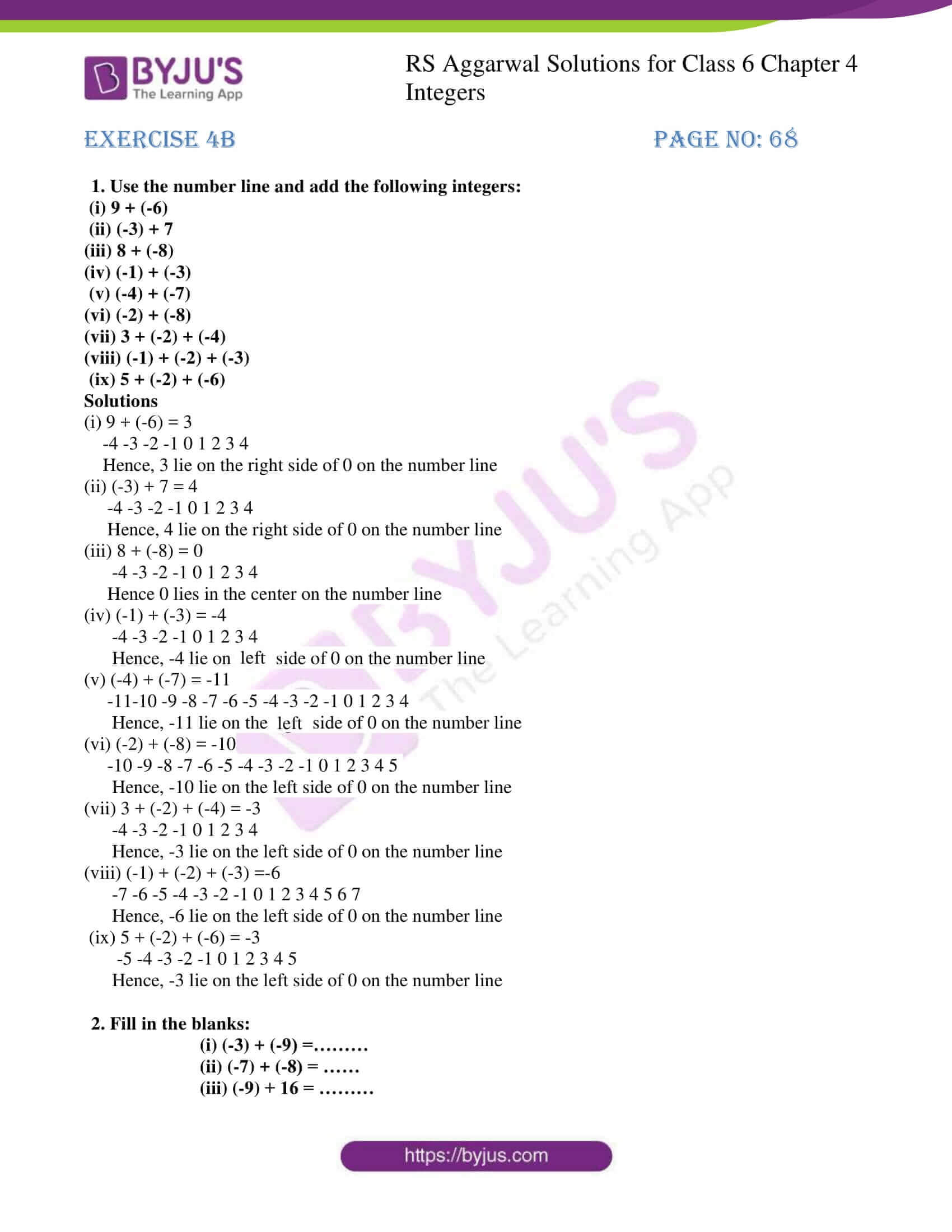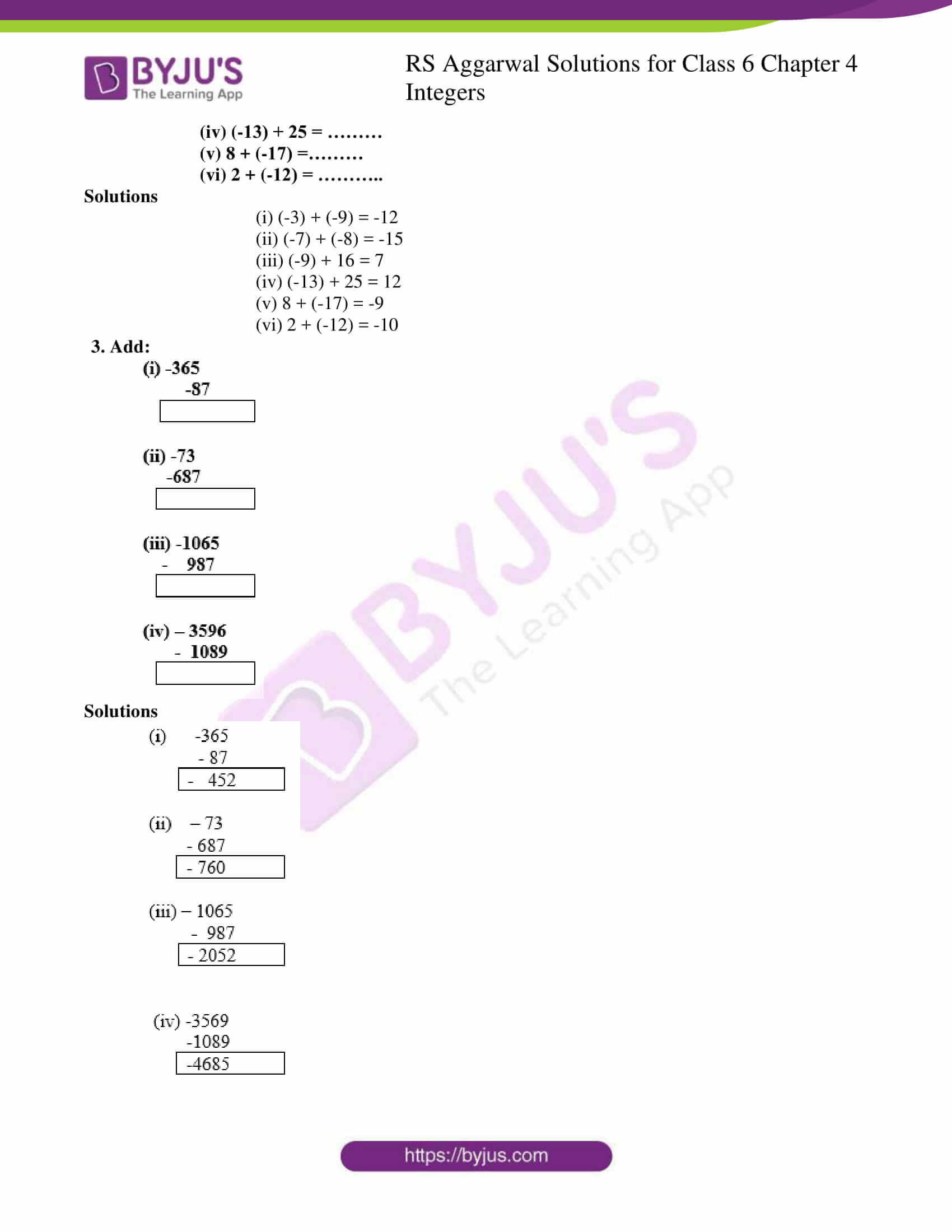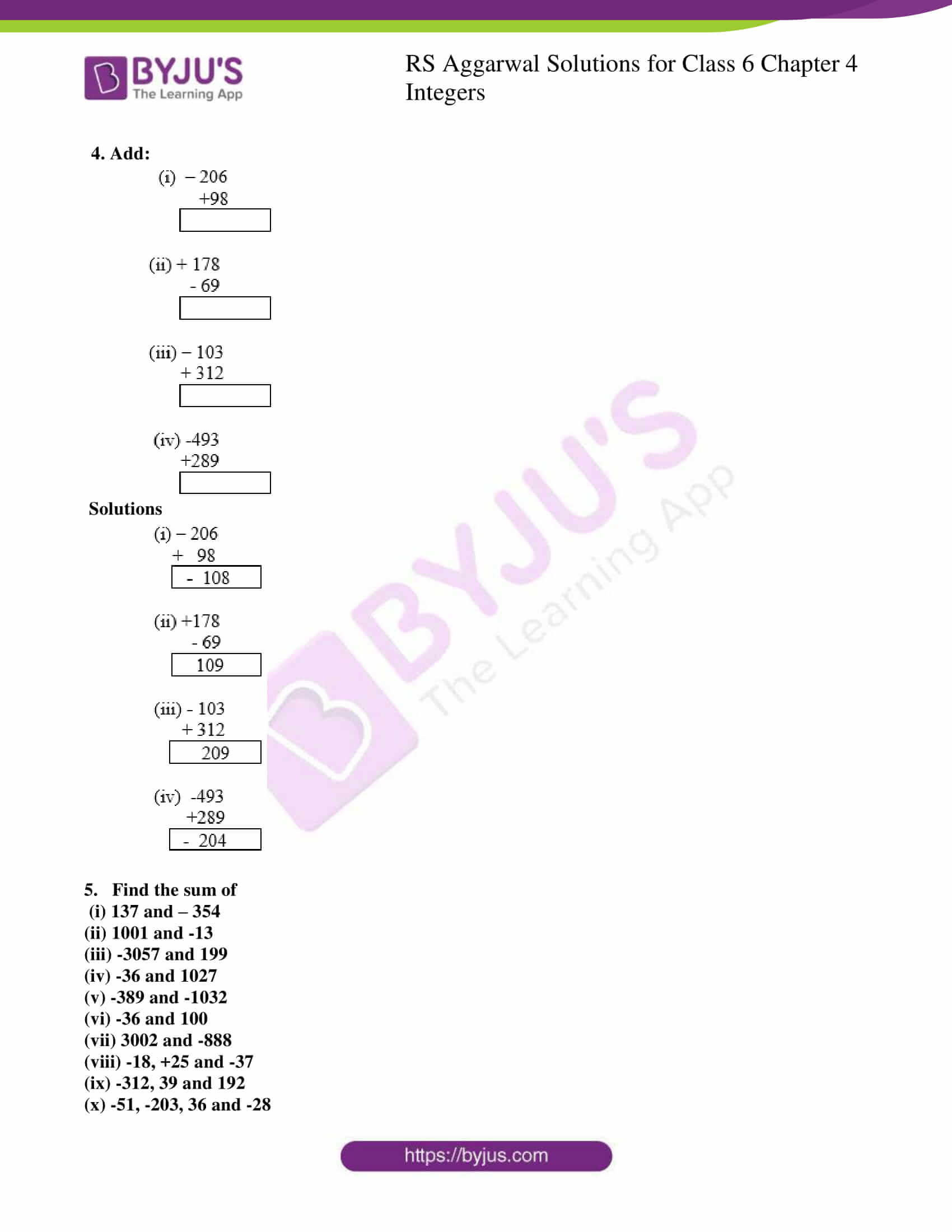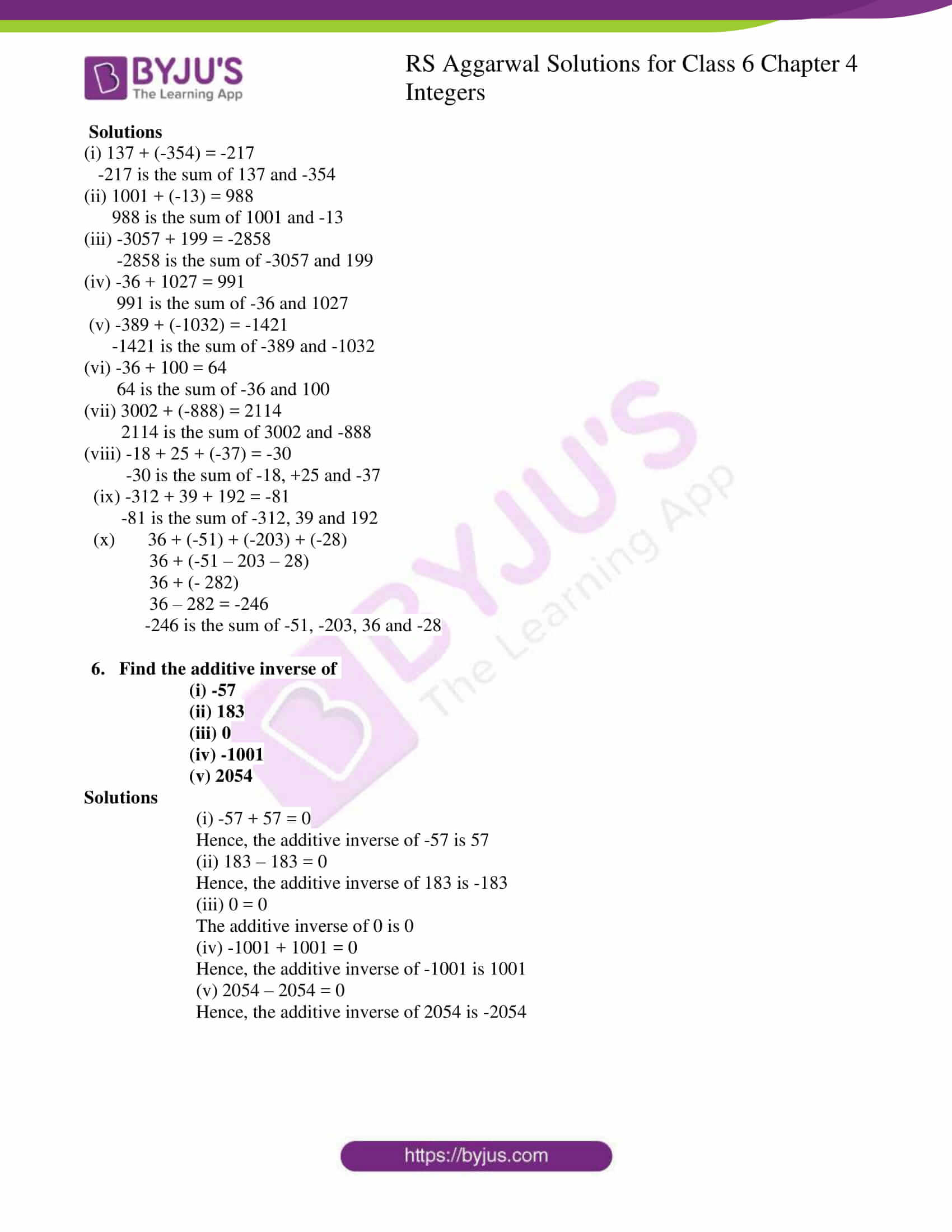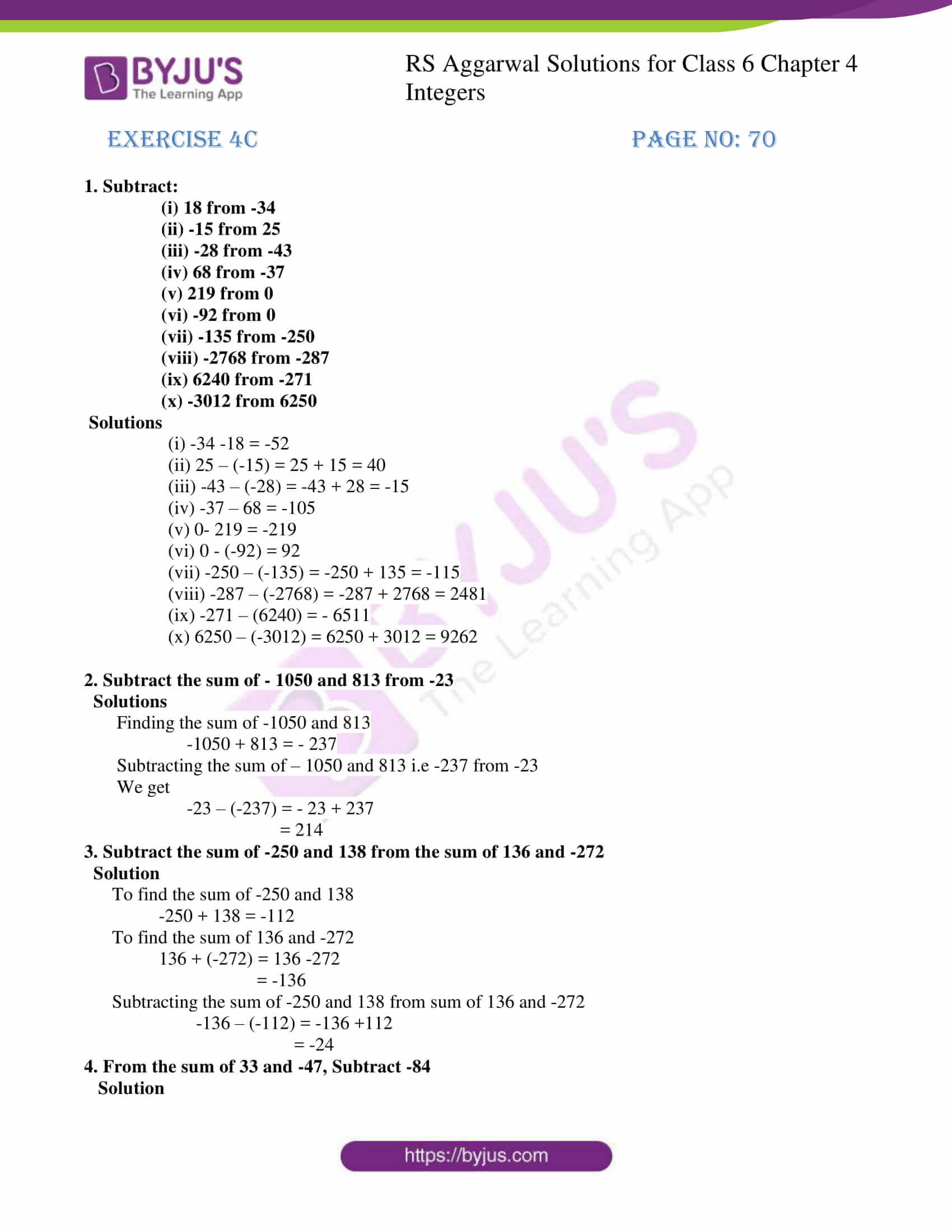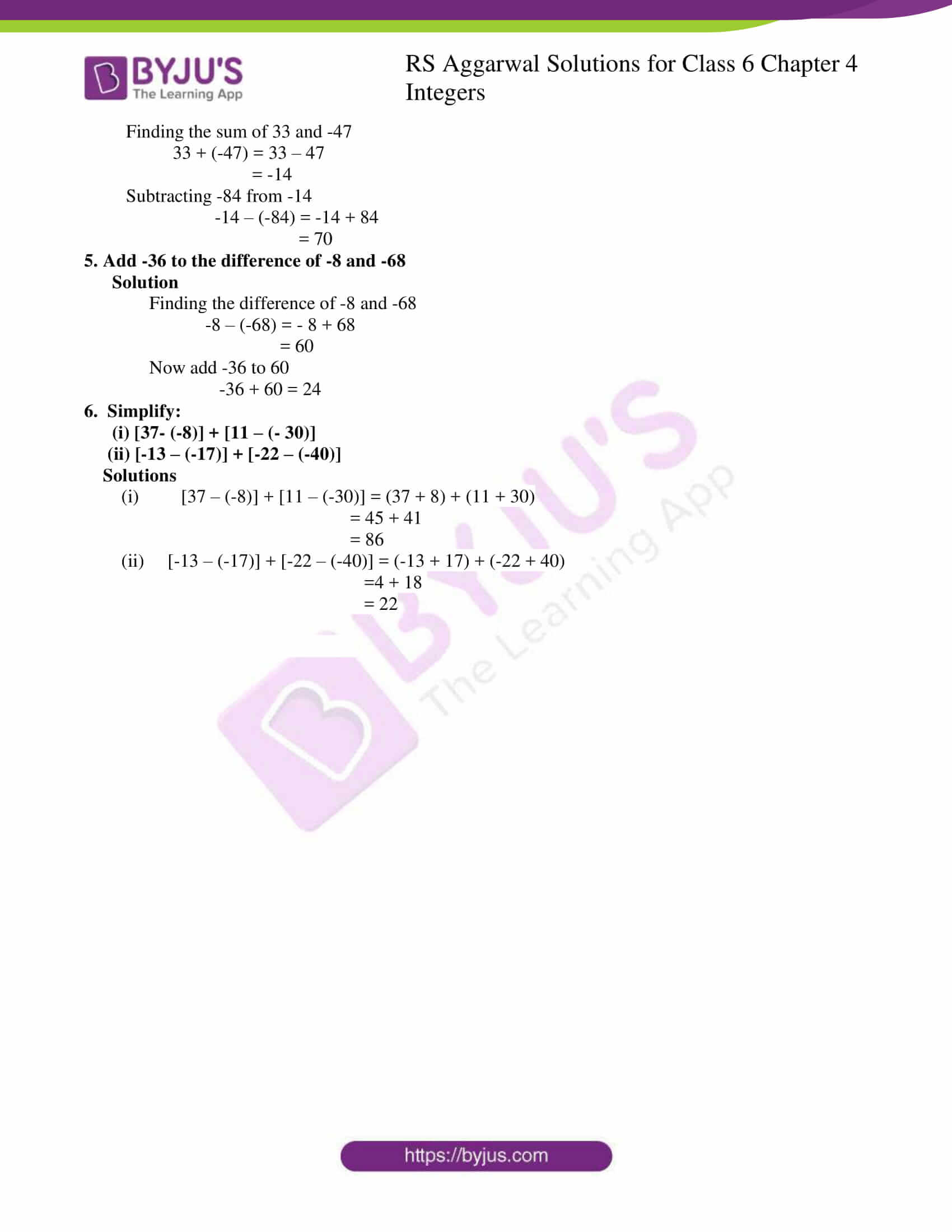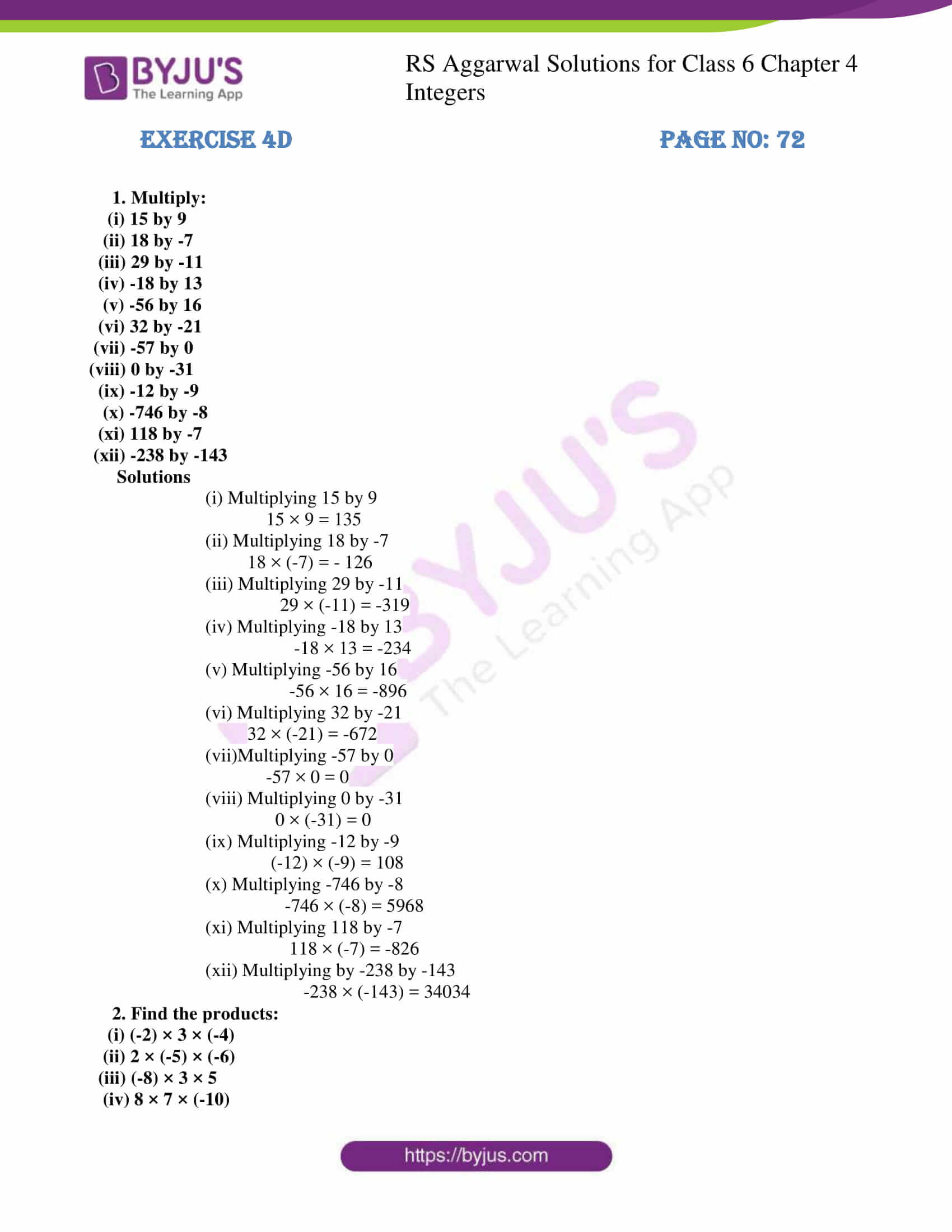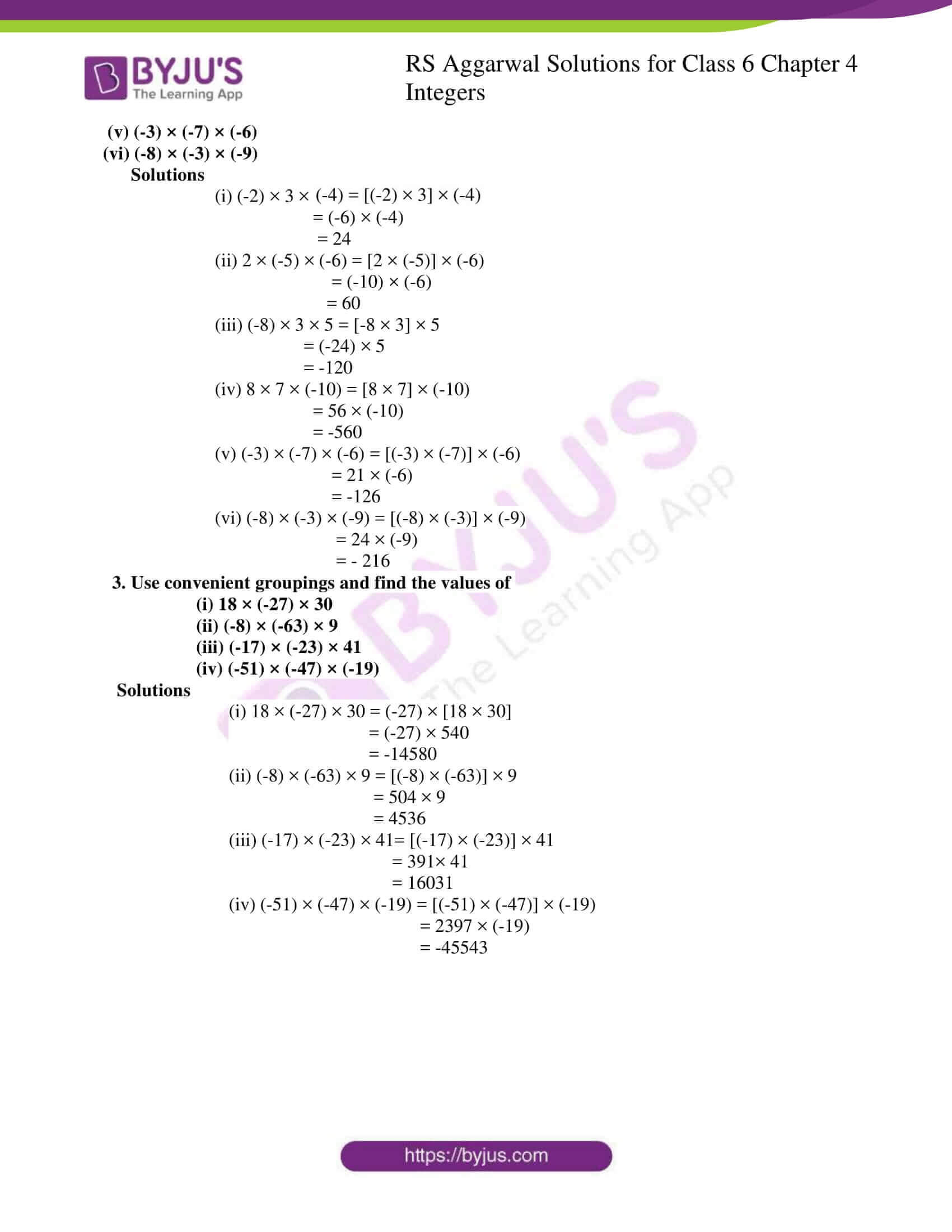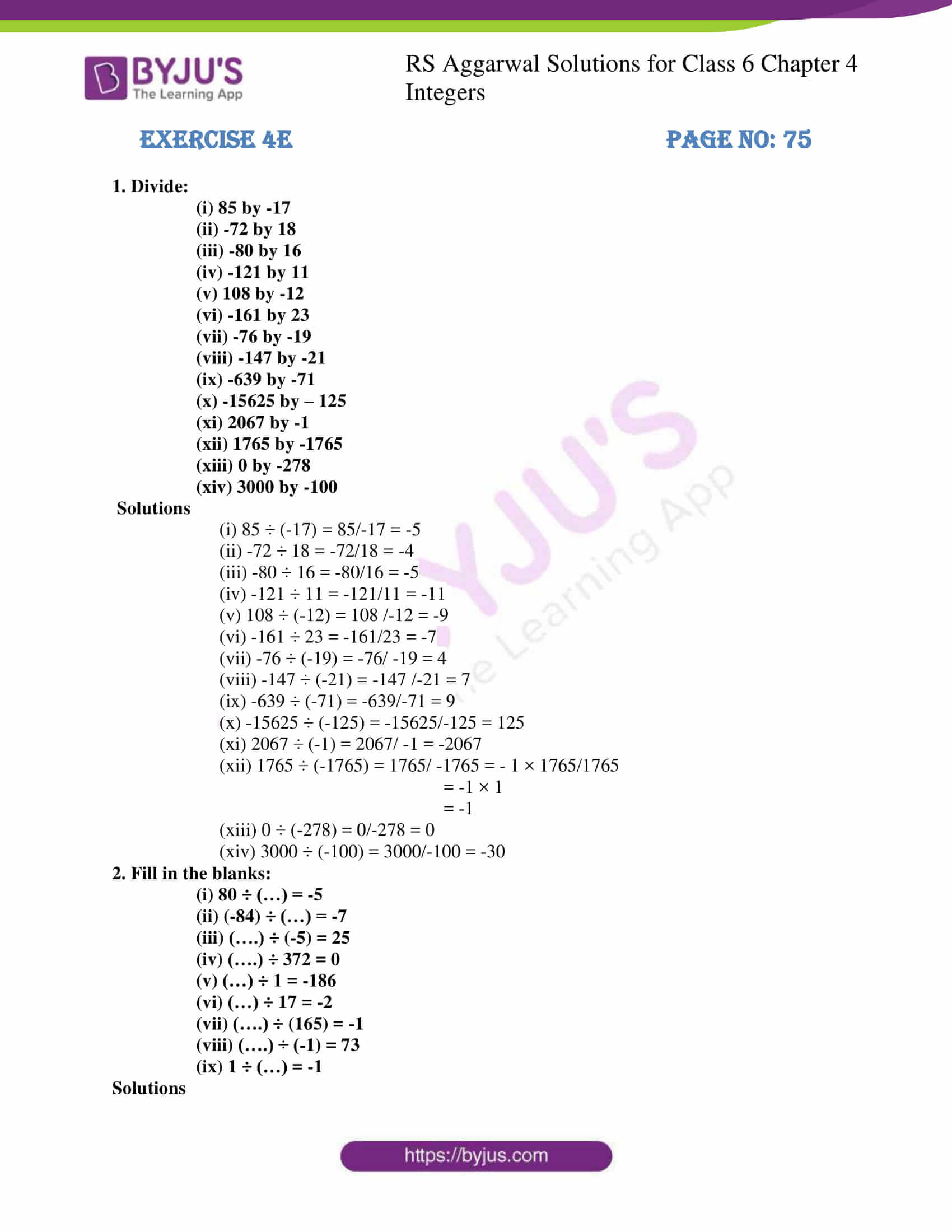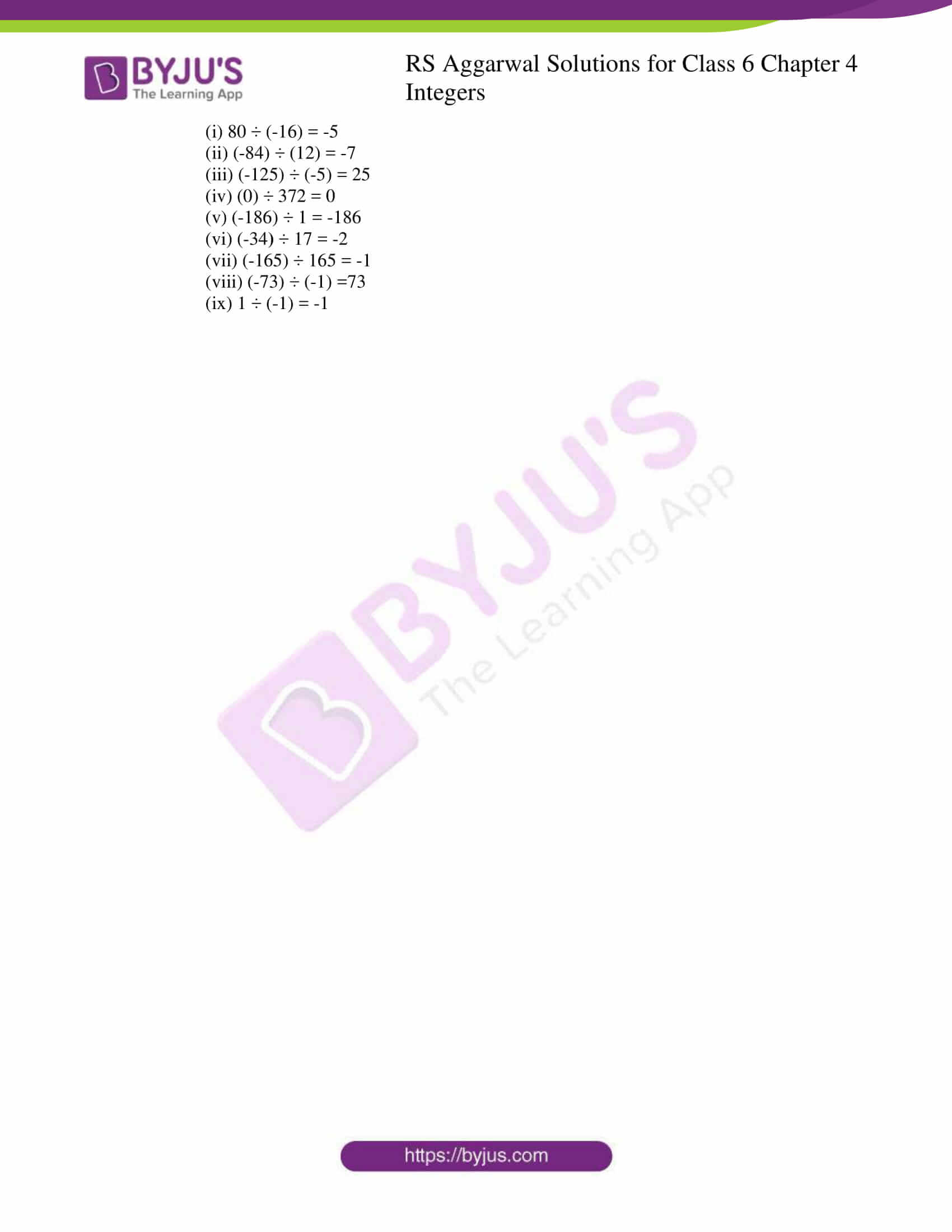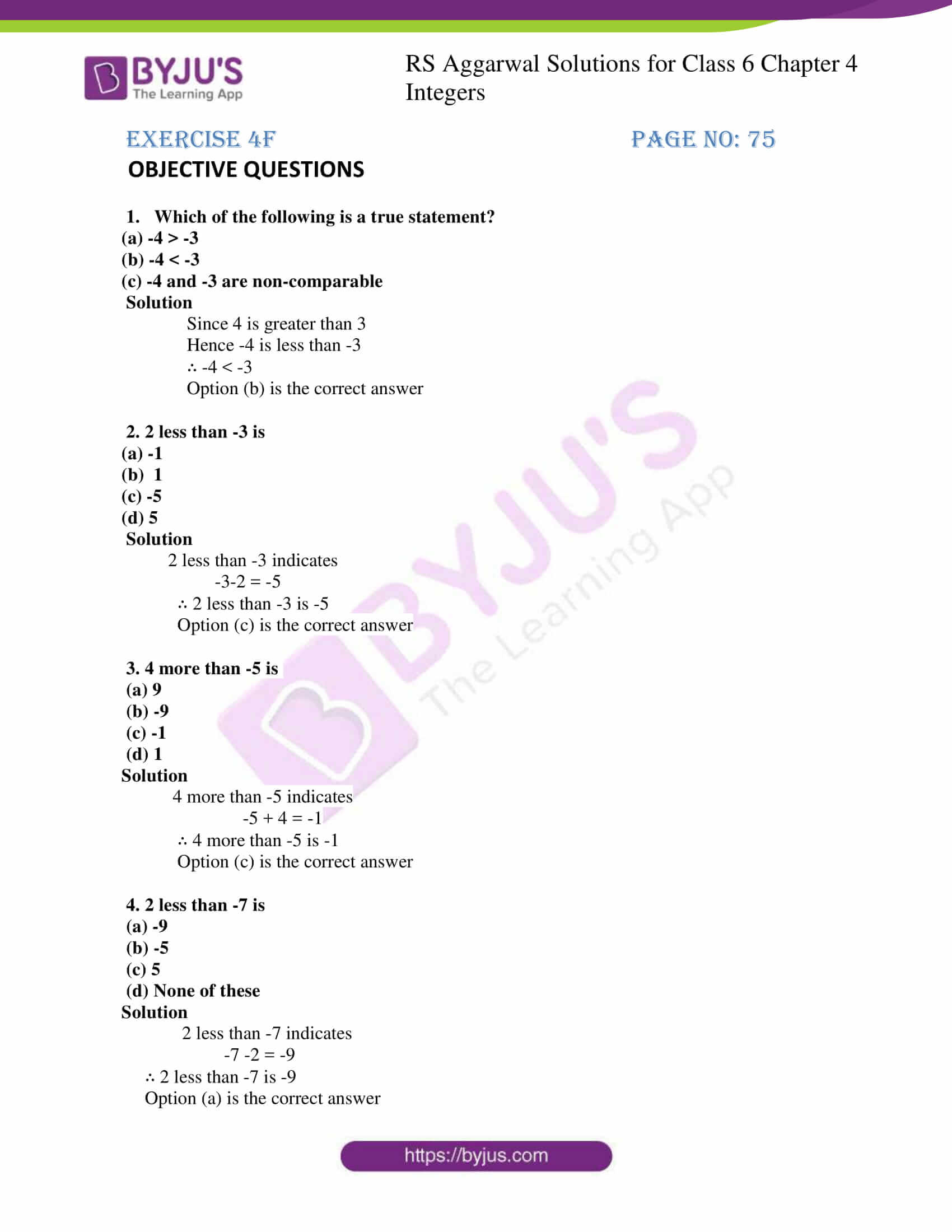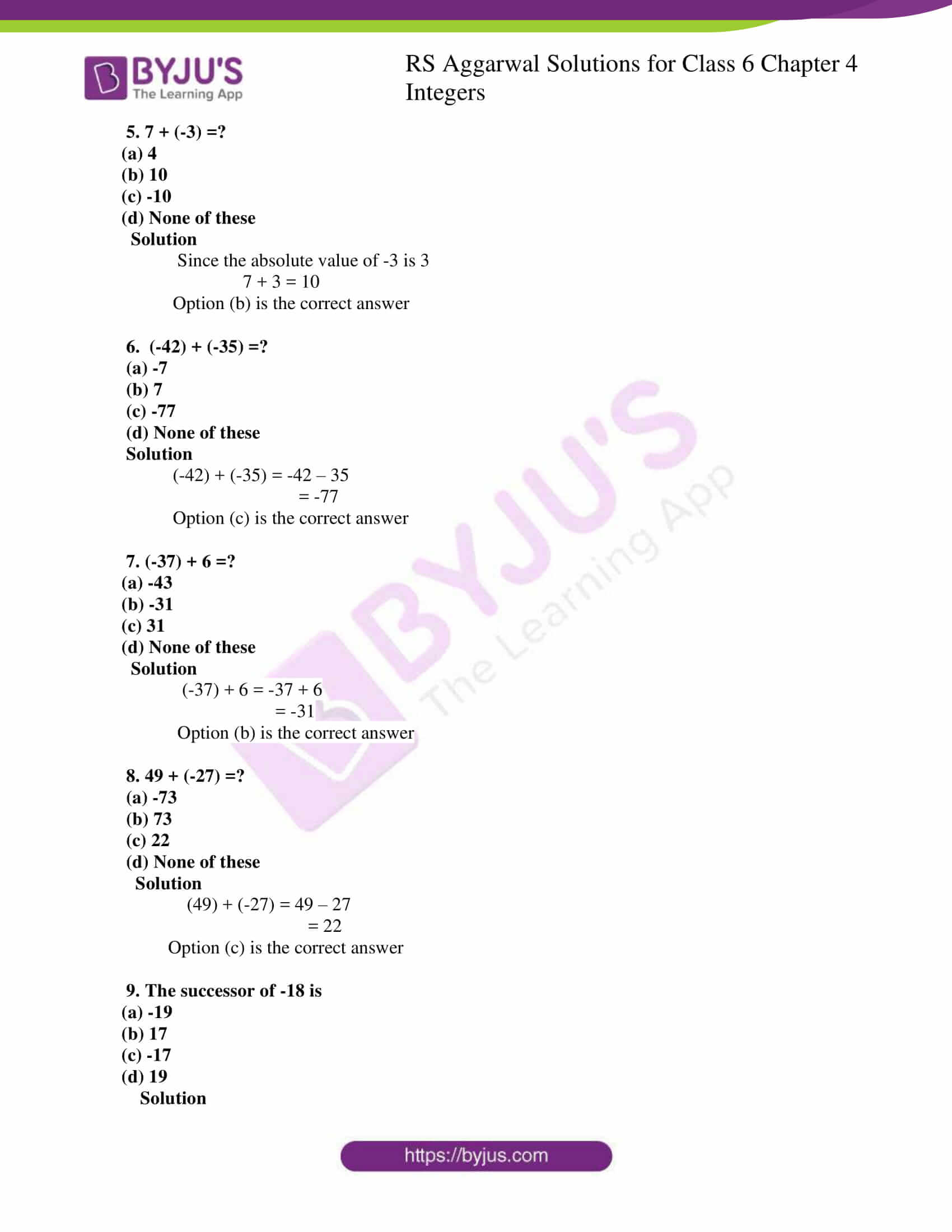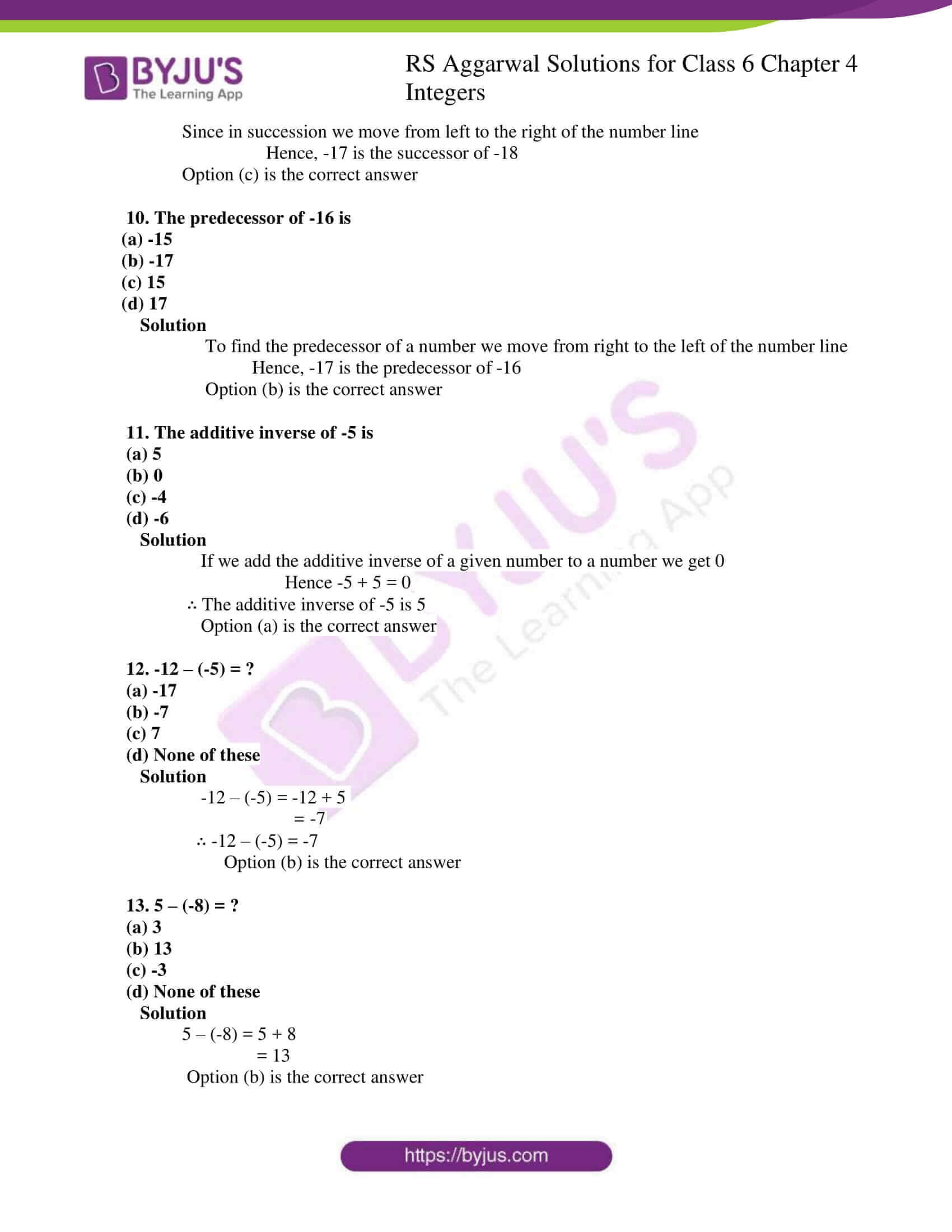## Exercise 4A PAGE NO: 63

1. Write the opposite of each of the following:

(i) An increase of 8 (ii) A loss of र 7

(iii) Gaining a weight of 5 kg (iv) 10 km above sea level

(v) 5oC below the freezing point (vi) A deposit of र 100

(vii) Earning र 500 (viii) Going 6 km to the east

(ix) 24 (x) -34

Solutions

The opposite statements

(i) The opposite statement of an increase of 8 is a decrease of 8

(ii) The opposite statement of a loss of र 7 is a gain of र 7

(iii) The opposite statement of gaining a weight of 5 kg is losing a weight of 5 kg

(iv) The opposite statement of 10 km above sea level is 10 km below sea level

(v) The opposite statement of 5oC below the freezing point is 5oC above the freezing point

(vi) The opposite statement of a deposit of र 100 is a withdrawal of र 100

(vii) The opposite statement of earning a र 500 is spending र 500

(viii) The opposite statement of going 6 km to the east is going 6 km to the west

(ix) The opposite of 24 is -24

(x) The opposite of -34 is 34

2. Indicate the following using “+” or “-“sign:

(i) A gain of र 600

(ii) A loss of र 800

(iii) 7oC below the freezing point

(iv) Decrease of 9

(v) 2 km above sea level

(vi) 3 km below sea level

(vii) A Deposit of र 200

(viii) A withdrawal of र 300

Solutions

(i) A gain of rupees 600 indicates +र 600

(ii) A loss of rupees 800 indicates -र 800

(iii) 7o C below the freezing point indicates -7oC

(iv) Decrease of 9 indicates -9

(v) 2 Km above sea level indicates +2 km

(vi) 3 km below sea level indicates –3 km

(vii) A deposit of rupees 200 indicates +र 200

(viii) A withdrawal of rupees 300 indicates -र 300

3. Mark the following integers on a number line:

(i) -5

(ii) -2

(iii) 0

(iv) 7

(v) -13

Solutions

(i) Here -5 lies on the left side of the 0 on the number line

-5 -4 -3 -2 -1 0 1 2 3 4 5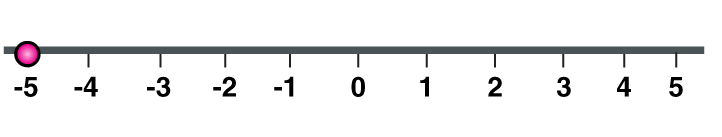(ii) Here -2 lies on the left side of the 0 on the number line

-5 -4 -3 -2 -1 0 1 2 3 4 5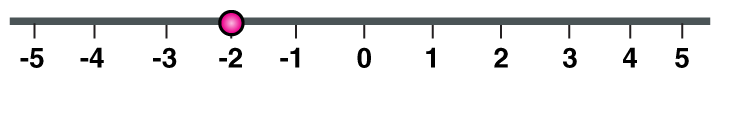(iii) Here 0 lies in the center on the number line

-5 -4 -3 -2 -1 0 1 2 3 4 5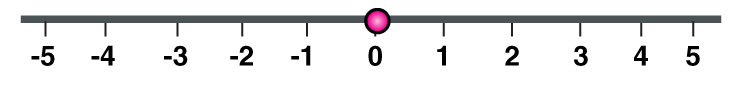(iv) Here 7 lies on the right side of the 0 on the number line

-7 -6 -5 -4 -3 -2 -1 0 1 2 3 4 5 6 7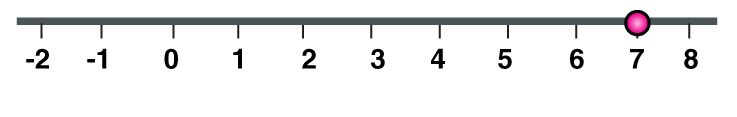(v) Here -13 lies on the left side of the 0 on the number line

-13 -12 -11 -10 -9 -8 -7 -6 -5 -4 -3 -2 -1 0 1 2 3 4 5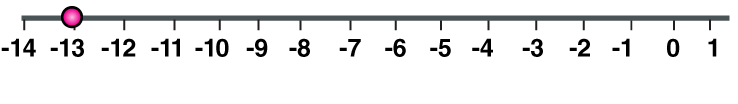4. Which number is larger in each of the following pairs?

(i) 0, -2

(ii) -3, -5

(iii) -5, 2

(iv) -16, 8

(v) -365, -913

(vi) -888, 8

Solutions

(i) Since 0 is greater than every negative integer

Hence 0 is greater than -2

(ii) Since 3 is less than 5

Hence -3 is greater than -5

(iii) Since every positive integer is greater than negative integer

Hence 2 is greater than -5

(iv) Since every positive integer is greater than negative integer

Hence 8 is greater than -16

(v) Since 365 is less than 913

Hence -365 is greater than -913

(vi) Since every positive integer is greater than negative integer

Hence 8 is greater than -888

5. Which number is smaller in each of the following pairs?

(i) 6, -7

(ii) 0, -1

(iii) -13, -27

(iv) -26, 17

(v) -317, -603

(vi) -777, 7

Solutions

(i) -7 is less than 6

(ii) -1 is less than 0

(iii) -27 is less than -13

(iv) -26 is less than 17

(v) -603 is less than -317

(vi) -777 is less than 7

6. Write all integers between

(i) 0 and 6 (ii) -5 and 0 (iii) -3 and 3 (iv) -7 and -5

Solutions

(i) 1, 2, 3, 4 and 5 are the integers between 0 and 6

(ii) -4, -3, -2, -1 are the integers between -5 and 0

(iii) -2, -1, 0, 1, and 2 are the integers between -3 and 3

(iv) -6 is the integer between -7 and -5

## Exercise 4B PAGE NO: 68

1. Use the number line and add the following integers:

(i) 9 + (-6)

(ii) (-3) + 7

(iii) 8 + (-8)

(iv) (-1) + (-3)

(v) (-4) + (-7)

(vi) (-2) + (-8)

(vii) 3 + (-2) + (-4)

(viii) (-1) + (-2) + (-3)

(ix) 5 + (-2) + (-6)

Solutions

(i) 9 + (-6) = 3

-4 -3 -2 -1 0 1 2 3 4

Hence, 3 lie on the right side of 0 on the number line

(ii) (-3) + 7 = 4

-4 -3 -2 -1 0 1 2 3 4

Hence, 4 lie on the right side of 0 on the number line

(iii) 8 + (-8) = 0

-4 -3 -2 -1 0 1 2 3 4

Hence 0 lies in the center on the number line

(iv) (-1) + (-3) = -4

-4 -3 -2 -1 0 1 2 3 4

Hence, -4 lie on left side of 0 on the number line

(v) (-4) + (-7) = -11

-11-10 -9 -8 -7 -6 -5 -4 -3 -2 -1 0 1 2 3 4

Hence, -11 lie on the left side of 0 on the number line

(vi) (-2) + (-8) = -10

-10 -9 -8 -7 -6 -5 -4 -3 -2 -1 0 1 2 3 4 5

Hence, -10 lie on the left side of 0 on the number line

(vii) 3 + (-2) + (-4) = -3

-4 -3 -2 -1 0 1 2 3 4

Hence, -3 lie on the left side of 0 on the number line

(viii) (-1) + (-2) + (-3) =-6

-7 -6 -5 -4 -3 -2 -1 0 1 2 3 4 5 6 7

Hence, -6 lie on the left side of 0 on the number line

(ix) 5 + (-2) + (-6) = -3

-5 -4 -3 -2 -1 0 1 2 3 4 5

Hence, -3 lie on the left side of 0 on the number line

2. Fill in the blanks:

(i) (-3) + (-9) =………

(ii) (-7) + (-8) = ……

(iii) (-9) + 16 = ………

(iv) (-13) + 25 = ………

(v) 8 + (-17) =………

(vi) 2 + (-12) = ………..

Solutions

(i) (-3) + (-9) = -12

(ii) (-7) + (-8) = -15

(iii) (-9) + 16 = 7

(iv) (-13) + 25 = 12

(v) 8 + (-17) = -9

(vi) 2 + (-12) = -10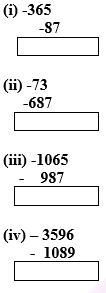Solutions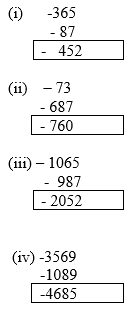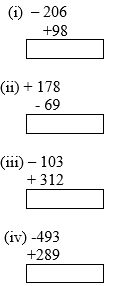Solutions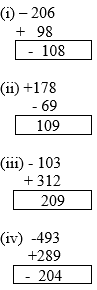5. Find the sum of

(i) 137 and – 354

(ii) 1001 and -13

(iii) -3057 and 199

(iv) -36 and 1027

(v) -389 and -1032

(vi) -36 and 100

(vii) 3002 and -888

(viii) -18, +25 and -37

(ix) -312, 39 and 192

(x) -51, -203, 36 and -28

Solutions

(i) 137 + (-354) = -217

-217 is the sum of 137 and -354

(ii) 1001 + (-13) = 988

988 is the sum of 1001 and -13

(iii) -3057 + 199 = -2858

-2858 is the sum of -3057 and 199

(iv) -36 + 1027 = 991

991 is the sum of -36 and 1027

(v) -389 + (-1032) = -1421

-1421 is the sum of -389 and -1032

(vi) -36 + 100 = 64

64 is the sum of -36 and 100

(vii) 3002 + (-888) = 2114

2114 is the sum of 3002 and -888

(viii) -18 + 25 + (-37) = -30

-30 is the sum of -18, +25 and -37

(ix) -312 + 39 + 192 = -81

-81 is the sum of -312, 39 and 192

(x) 36 + (-51) + (-203) + (-28)

36 + (-51 – 203 – 28)

36 + (- 282)

36 – 282 = -246

-246 is the sum of -51, -203, 36 and -28

6. Find the additive inverse of

(i) -57

(ii) 183

(iii) 0

(iv) -1001

(v) 2054

Solutions

(i) -57 + 57 = 0

Hence, the additive inverse of -57 is 57

(ii) 183 – 183 = 0

Hence, the additive inverse of 183 is -183

(iii) 0 = 0

The additive inverse of 0 is 0

(iv) -1001 + 1001 = 0

Hence, the additive inverse of -1001 is 1001

(v) 2054 – 2054 = 0

Hence, the additive inverse of 2054 is -2054

## Exercise 4C PAGE NO: 70

1. Subtract:

(i) 18 from -34

(ii) -15 from 25

(iii) -28 from -43

(iv) 68 from -37

(v) 219 from 0

(vi) -92 from 0

(vii) -135 from -250

(viii) -2768 from -287

(ix) 6240 from -271

(x) -3012 from 6250

Solutions

(i) -34 -18 = -52

(ii) 25 – (-15) = 25 + 15 = 40

(iii) -43 – (-28) = -43 + 28 = -15

(iv) -37 – 68 = -105

(v) 0- 219 = -219

(vi) 0 – (-92) = 92

(vii) -250 – (-135) = -250 + 135 = -115

(viii) -287 – (-2768) = -287 + 2768 = 2481

(ix) -271 – (6240) = – 6511

(x) 6250 – (-3012) = 6250 + 3012 = 9262

2. Subtract the sum of – 1050 and 813 from -23

Solutions

Finding the sum of -1050 and 813

-1050 + 813 = – 237

Subtracting the sum of – 1050 and 813 i.e -237 from -23

We get

-23 – (-237) = – 23 + 237

= 214

3. Subtract the sum of -250 and 138 from the sum of 136 and -272

Solution

To find the sum of -250 and 138

-250 + 138 = -112

To find the sum of 136 and -272

136 + (-272) = 136 -272

= -136

Subtracting the sum of -250 and 138 from sum of 136 and -272

-136 – (-112) = -136 +112

= -24

4. From the sum of 33 and -47, Subtract -84

Solution

Finding the sum of 33 and -47

33 + (-47) = 33 – 47

= -14

Subtracting -84 from -14

-14 – (-84) = -14 + 84

= 70

5. Add -36 to the difference of -8 and -68

Solution

Finding the difference of -8 and -68

-8 – (-68) = – 8 + 68

= 60

-36 + 60 = 24

6. Simplify:

(i) [37- (-8)] + [11 – (- 30)]

(ii) [-13 – (-17)] + [-22 – (-40)]

Solutions

(i) [37 – (-8)] + [11 – (-30)] = (37 + 8) + (11 + 30)

= 45 + 41

= 86

(ii) [-13 – (-17)] + [-22 – (-40)] = (-13 + 17) + (-22 + 40)

=4 + 18

= 22

## Exercise 4D PAGE NO: 72

1. Multiply:

(i) 15 by 9

(ii) 18 by -7

(iii) 29 by -11

(iv) -18 by 13

(v) -56 by 16

(vi) 32 by -21

(vii) -57 by 0

(viii) 0 by -31

(ix) -12 by -9

(x) -746 by -8

(xi) 118 by -7

(xii) -238 by -143

Solutions

(i) Multiplying 15 by 9

15 × 9 = 135

(ii) Multiplying 18 by -7

18 × (-7) = – 126

(iii) Multiplying 29 by -11

29 × (-11) = -319

(iv) Multiplying -18 by 13

-18 × 13 = -234

(v) Multiplying -56 by 16

-56 × 16 = -896

(vi) Multiplying 32 by -21

32 × (-21) = -672

(vii)Multiplying -57 by 0

-57 × 0 = 0

(viii) Multiplying 0 by -31

0 × (-31) = 0

(ix) Multiplying -12 by -9

(-12) × (-9) = 108

(x) Multiplying -746 by -8

-746 × (-8) = 5968

(xi) Multiplying 118 by -7

118 × (-7) = -826

(xii) Multiplying by -238 by -143

-238 × (-143) = 34034

2. Find the products:

(i) (-2) × 3 × (-4)

(ii) 2 × (-5) × (-6)

(iii) (-8) × 3 × 5

(iv) 8 × 7 × (-10)

(v) (-3) × (-7) × (-6)

(vi) (-8) × (-3) × (-9)

Solutions

(i) (-2) × 3 × (-4) = [(-2) × 3] × (-4)

= (-6) × (-4)

= 24

(ii) 2 × (-5) × (-6) = [2 × (-5)] × (-6)

= (-10) × (-6)

= 60

(iii) (-8) × 3 × 5 = [-8 × 3] × 5

= (-24) × 5

= -120

(iv) 8 × 7 × (-10) = [8 × 7] × (-10)

= 56 × (-10)

= -560

(v) (-3) × (-7) × (-6) = [(-3) × (-7)] × (-6)

= 21 × (-6)

= -126

(vi) (-8) × (-3) × (-9) = [(-8) × (-3)] × (-9)

= 24 × (-9)

= – 216

3. Use convenient groupings and find the values of

(i) 18 × (-27) × 30

(ii) (-8) × (-63) × 9

(iii) (-17) × (-23) × 41

(iv) (-51) × (-47) × (-19)

Solutions

(i) 18 × (-27) × 30 = (-27) × [18 × 30]

= (-27) × 540

= -14580

(ii) (-8) × (-63) × 9 = [(-8) × (-63)] × 9

= 504 × 9

= 4536

(iii) (-17) × (-23) × 41= [(-17) × (-23)] × 41

= 391× 41

= 16031

(iv) (-51) × (-47) × (-19) = [(-51) × (-47)] × (-19)

= 2397 × (-19)

= -45543

## Exercise 4E PAGE NO: 75

1. Divide:

(i) 85 by -17

(ii) -72 by 18

(iii) -80 by 16

(iv) -121 by 11

(v) 108 by -12

(vi) -161 by 23

(vii) -76 by -19

(viii) -147 by -21

(ix) -639 by -71

(x) -15625 by – 125

(xi) 2067 by -1

(xii) 1765 by -1765

(xiii) 0 by -278

(xiv) 3000 by -100

Solutions

(i) 85 ÷ (-17) = 85/-17 = -5

(ii) -72 ÷ 18 = -72/18 = -4

(iii) -80 ÷ 16 = -80/16 = -5

(iv) -121 ÷ 11 = -121/11 = -11

(v) 108 ÷ (-12) = 108 /-12 = -9

(vi) -161 ÷ 23 = -161/23 = -7

(vii) -76 ÷ (-19) = -76/ -19 = 4

(viii) -147 ÷ (-21) = -147 /-21 = 7

(ix) -639 ÷ (-71) = -639/-71 = 9

(x) -15625 ÷ (-125) = -15625/-125 = 125

(xi) 2067 ÷ (-1) = 2067/ -1 = -2067

(xii) 1765 ÷ (-1765) = 1765/ -1765 = – 1 × 1765/1765

= -1 × 1

= -1

(xiii) 0 ÷ (-278) = 0/-278 = 0

(xiv) 3000 ÷ (-100) = 3000/-100 = -30

2. Fill in the blanks:

(i) 80 ÷ (…) = -5

(ii) (-84) ÷ (…) = -7

(iii) (….) ÷ (-5) = 25

(iv) (….) ÷ 372 = 0

(v) (…) ÷ 1 = -186

(vi) (…) ÷ 17 = -2

(vii) (….) ÷ (165) = -1

(viii) (….) ÷ (-1) = 73

(ix) 1 ÷ (…) = -1

Solutions

(i) 80 ÷ (-16) = -5

(ii) (-84) ÷ (12) = -7

(iii) (-125) ÷ (-5) = 25

(iv) (0) ÷ 372 = 0

(v) (-186) ÷ 1 = -186

(vi) (-34) ÷ 17 = -2

(vii) (-165) ÷ 165 = -1

(viii) (-73) ÷ (-1) =73

(ix) 1 ÷ (-1) = -1

## Exercise 4F PAGE NO: 75

OBJECTIVE QUESTIONS

1. Which of the following is a true statement?

(a) -4 > -3

(b) -4 < -3

(c) -4 and -3 are non-comparable

Solution

Since 4 is greater than 3

Hence -4 is less than -3

∴ -4 < -3

Option (b) is the correct answer

2. 2 less than -3 is

(a) -1

(b) 1

(c) -5

(d) 5

Solution

2 less than -3 indicates

-3-2 = -5

∴ 2 less than -3 is -5

Option (c) is the correct answer

3. 4 more than -5 is

(a) 9

(b) -9

(c) -1

(d) 1

Solution

4 more than -5 indicates

-5 + 4 = -1

∴ 4 more than -5 is -1

Option (c) is the correct answer

4. 2 less than -7 is

(a) -9

(b) -5

(c) 5

(d) None of these

Solution

2 lessthan -7 indicates

-7 -2 = -9

∴ 2 less than -7 is -9

Option (a) is the correct answer

5. 7 + (-3) =?

(a) 4

(b) 10

(c) -10

(d) None of these

Solution

Since the absolute value of -3 is 3

7 + 3 = 10

Option (b) is the correct answer

6. (-42) + (-35) =?

(a) -7

(b) 7

(c) -77

(d) None of these

Solution

(-42) + (-35) = -42 – 35

= -77

Option (c) is the correct answer

7. (-37) + 6 =?

(a) -43

(b) -31

(c) 31

(d) None of these

Solution

(-37) + 6 = -37 + 6

= -31

Option (b) is the correct answer

8. 49 + (-27) =?

(a) -73

(b) 73

(c) 22

(d) None of these

Solution

(49) + (-27) = 49 – 27

= 22

Option (c) is the correct answer

9. The successor of -18 is

(a) -19

(b) 17

(c) -17

(d) 19

Solution

Since in succession we move from left to the right of the number line

Hence, -17 is the successor of -18

Option (c) is the correct answer

10. The predecessor of -16 is

(a) -15

(b) -17

(c) 15

(d) 17

Solution

To find the predecessor of a number we move from right to the left of the number line

Hence, -17 is the predecessor of -16

Option (b) is the correct answer

11. The additive inverse of -5 is

(a) 5

(b) 0

(c) -4

(d) -6

Solution

If we add the additive inverse of a given number to a number we get 0

Hence -5 + 5 = 0

∴ The additive inverse of -5 is 5

Option (a) is the correct answer

12. -12 – (-5) = ?

(a) -17

(b) -7

(c) 7

(d) None of these

Solution

-12 – (-5) = -12 + 5

= – 7

∴ -12 – (-5) = -7

Option (b) is the correct answer

13. 5 – (-8) = ?

(a) 3

(b) 13

(c) -3

(d) None of these

Solution

5 – (-8) = 5 + 8

= 13

Option (b) is the correct answer

### RS Aggarwal Solutions for Class 6 Maths Chapter 4 Integers

Chapter 4 – Integers consists of 6 exercises. RS Aggarwal Solutions are solved in detail for each question in every exercise. Let’s have a glance at the topics mentioned in this Chapter.

• Representation of integers on the number line
• Operations on integers
• Properties of addition on integers
• Subtraction of integers
• Properties of subtraction on integers
• Multiplication of integers
• Properties of multiplication on integers
• Division on integers
• Properties of division on integers

### Chapter Brief of RS Aggarwal Solutions for Class 6 Maths Chapter 4 Integers

The set of numbers containing whole numbers and their opposites are called integers.

Integers are either positive or negative. Positive integers are placed at the right side of the number line and negative integers are at the left side of the number line. 0, lies at the centre which is neither positive nor negative. Integers are used in clocks, thermometers, addresses and money.